# Normal and Probability Distribution

Normal distribution is a continuous probability distribution. It is also called Gaussian distribution.
The normal distribution density function f(z) is called the Bell Curve because it has the shape that resembles a bell.
Standard normal distribution table is used to find the area under the f(z) function in order to find the probability of a specified range of distribution.

Normal distribution function

When random variable X has normal distribution,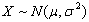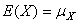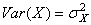The probability density function and cumulative distribution function of the normal distribution:

#### Probability density function (pdf)

The probability density function is given by: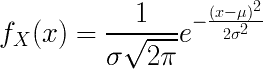X is the random variable.
μ is the mean value.
σ is the standard deviation (std) value.
e = 2.7182818… constant.
π = 3.1415926… constant.

#### Cumulative distribution function

The cumulative distribution function is given by: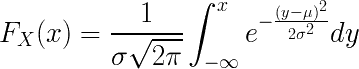X is the random variable.

μ is the mean value.

σ is the standard deviation (std) value.

e = 2.7182818… constant.

π = 3.1415926… constant.

Standard normal distribution function

When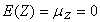Then the probability density function and cumulative distribution function of the standard normal distribution:

#### Probability density function#### Cumulative distribution function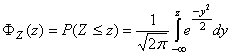In probability and statistics distribution is a characteristic of a random variable, describes the probability of the random variable in each value.

Each distribution has a certain probability density function and probability distribution function.

Though there are indefinite number of probability distributions, there are several common distributions in use.

Cumulative distribution function

The probability distribution is described by the cumulative distribution function F(x),

which is the probability of random variable X to get value smaller than or equal to x:

F(x) = P(Xx)

The cumulative distribution function F(x) is calculated by integration of the probability density function f(u) of continuous random variable X.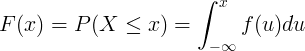The cumulative distribution function F(x) is calculated by summation of the probability mass function P(u) of discrete random variable X.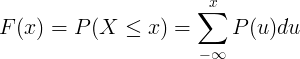Continuous distribution is the distribution of a continuous random variable

#### Continuous distributions table

Distribution name
Distribution symbol
Probability density function (pdf)
Mean
Variance

fX(x)

μ = E(X)

σ2 = Var(X)

Normal / gaussian

X ~ N(μ,σ2)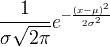μ
σ2
Uniform

X ~ U(a,b)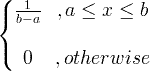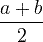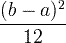Exponential
X ~ exp(λ)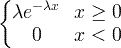Gamma
X ~ gamma(c, λ)x > 0, c > 0, λ > 0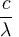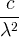Chi square

X 2(k)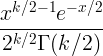k

2k

F

X ~ F (k1, k2)

Log-normal

X ~ LN(μ,σ2)

Discrete distribution is the distribution of a discrete random variable.

#### Discrete distributions table

Distribution name
Distribution symbol
Probability mass function (pmf)
Mean
Variance

fx(k) = P(X=k)

k = 0,1,2,…

E(x)
Var(x)
Binomial
X ~ Bin(n,p)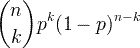np
np(1-p)
Poisson
X ~ Poisson(λ)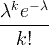λ ≥ 0

λ
λ
Uniform
X ~ U(a,b)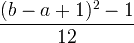Geometric
X ~ Geom(p)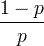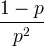Hyper-geometric
X ~ HG(N,K,n)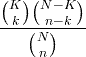N = 0,1,2,…

K = 0,1,..,N

n = 0,1,…,N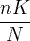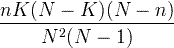Bernoulli
X ~ Bern(p)p
p(1-p)